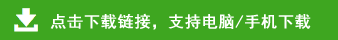# 小学一年级数学口算+应用题专项练习

75+27=    68-19=    38+59=    78-49=
67+25=    57-38=    46+46=    64-57=
29+47=    67-59=    16+67=    54-48=
54+46=    71-65=    54+36=    48-39=
49+49=    91-85=    36+14=    25+41=
79-64=    19+21=    11+9＝    15-6＝
32+2＝    50＋1＝    30＋2＝    16-9＝